### Algorithm Descriptions

#### Trust Region Optimization (TRUREG)

The trust region method uses the gradient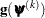and the Hessian matrix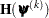; thus, it requires that the objective functionhave continuous first- and second-order derivatives inside the feasible region.

The trust region method iteratively optimizes a quadratic approximation to the nonlinear objective function within a hyperelliptic trust region with radius
that constrains the step size that corresponds to the quality of the quadratic approximation. The trust region method is implemented based on Dennis, Gay, and Welsch (1981), Gay (1983), and Moré and Sorensen (1983).

The trust region method performs well for small- to medium-sized problems, and it does not need many function, gradient, and Hessian calls. However, if the computation of the Hessian matrix is computationally expensive, one of the (dual) quasi-Newton or conjugate gradient algorithms might be more efficient.

#### Newton-Raphson Optimization with Line Search (NEWRAP)

The NEWRAP technique uses the gradientand the Hessian matrix; thus, it requires that the objective function have continuous first- and second-order derivatives inside the feasible region. If second-order derivatives are computed efficiently and precisely, the NEWRAP method can perform well for medium-sized to large problems, and it does not need many function, gradient, and Hessian calls.

This algorithm uses a pure Newton step when the Hessian is positive definite and when the Newton step reduces the value of the objective function successfully. Otherwise, a combination of ridging and line search is performed to compute successful steps. If the Hessian is not positive definite, a multiple of the identity matrix is added to the Hessian matrix to make it positive definite (Eskow and Schnabel 1991).

In each iteration, a line search is performed along the search direction to find an approximate optimum of the objective function. The default line-search method uses quadratic interpolation and cubic extrapolation (LIS=2).

#### Newton-Raphson Ridge Optimization (NRRIDG)

The NRRIDG technique uses the gradientand the Hessian matrix; thus, it requires that the objective function have continuous first- and second-order derivatives inside the feasible region.

This algorithm uses a pure Newton step when the Hessian is positive definite and when the Newton step reduces the value of the objective function successfully. If at least one of these two conditions is not satisfied, a multiple of the identity matrix is added to the Hessian matrix.

The NRRIDG method performs well for small- to medium-sized problems, and it does not require many function, gradient, and Hessian calls. However, if the computation of the Hessian matrix is computationally expensive, one of the (dual) quasi-Newton or conjugate gradient algorithms might be more efficient.

Because the NRRIDG technique uses an orthogonal decomposition of the approximate Hessian, each iteration of NRRIDG can be slower than that of the NEWRAP technique, which works with a Cholesky decomposition. Usually, however, NRRIDG requires fewer iterations than NEWRAP.

#### Quasi-Newton Optimization (QUANEW)

The (dual) quasi-Newton method uses the gradient, and it does not need to compute second-order derivatives because they are approximated. It works well for medium-sized to moderately large optimization problems, where the objective function and the gradient are much faster to compute than the Hessian. However, in general, it requires more iterations than the TRUREG, NEWRAP, and NRRIDG techniques, which compute second-order derivatives. QUANEW is the default optimization algorithm because it provides an appropriate balance between the speed and stability required for most nonlinear mixed model applications.

The QUANEW technique is one of the following, depending upon the value of the UPDATE= option:

• the original quasi-Newton algorithm, which updates an approximation of the inverse Hessian

• the dual quasi-Newton algorithm, which updates the Cholesky factor of an approximate Hessian (this is the default)

You can specify four update formulas with the UPDATE= option:

• DBFGS performs the dual Broyden, Fletcher, Goldfarb, and Shanno (BFGS) update of the Cholesky factor of the Hessian matrix. This is the default.

• DDFP performs the dual Davidon, Fletcher, and Powell (DFP) update of the Cholesky factor of the Hessian matrix.

• BFGS performs the original BFGS update of the inverse Hessian matrix.

• DFP performs the original DFP update of the inverse Hessian matrix.

In each iteration, a line search is performed along the search direction to find an approximate optimum. The default line-search method uses quadratic interpolation and cubic extrapolation to obtain a step sizethat satisfies the Goldstein conditions. One of the Goldstein conditions can be violated if the feasible region defines an upper limit of the step size. Violating the left-side Goldstein condition can affect the positive definiteness of the quasi-Newton update. In that case, either the update is skipped or the iterations are restarted with an identity matrix, resulting in the steepest descent or ascent search direction. You can specify line-search algorithms other than the default with the LIS= option.

The QUANEW algorithm uses its own line-search technique. Of the options and parameters that control the line search for other algorithms, only the INSTEP= option applies here. In several applications, large steps in the first iterations are troublesome. You can use the INSTEP= option to impose an upper bound for the step sizeduring the first five iterations. You can also use the INHESSIAN= option to specify a different starting approximation for the Hessian. If you specify only the INHESSIAN option, the Cholesky factor of a (possibly ridged) finite-difference approximation of the Hessian is used to initialize the quasi-Newton update process.

#### Double-Dogleg Optimization (DBLDOG)

The double-dogleg optimization method combines the ideas of the quasi-Newton and trust region methods. In each iteration, the double-dogleg algorithm computes the step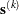as the linear combination of the steepest descent or ascent search direction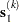and a quasi-Newton search direction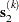,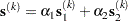The step is requested to remain within a prespecified trust region radius; see Fletcher (1987, p. 107). Thus, the DBLDOG subroutine uses the dual quasi-Newton update but does not perform a line search. You can specify two update formulas with the UPDATE= option:

• DBFGS performs the dual Broyden, Fletcher, Goldfarb, and Shanno update of the Cholesky factor of the Hessian matrix. This is the default.

• DDFP performs the dual Davidon, Fletcher, and Powell update of the Cholesky factor of the Hessian matrix.

The double-dogleg optimization technique works well for medium-sized to moderately large optimization problems, where the objective function and the gradient are much faster to compute than the Hessian. The implementation is based on Dennis and Mei (1979) and Gay (1983), but it is extended for dealing with boundary and linear constraints. The DBLDOG technique generally requires more iterations than the TRUREG, NEWRAP, and NRRIDG techniques, which require second-order derivatives; however, each of the DBLDOG iterations is computationally cheap. Furthermore, the DBLDOG technique requires only gradient calls for the update of the Cholesky factor of an approximate Hessian.

Second-order derivatives are not required by the CONGRA algorithm and are not even approximated. The CONGRA algorithm can be expensive in function and gradient calls, but it requires only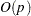memory for unconstrained optimization. In general, many iterations are required to obtain a precise solution, but each of the CONGRA iterations is computationally cheap. You can specify four different update formulas for generating the conjugate directions by using the UPDATE= option:

• PB performs the automatic restart update method of Powell (1977) and Beale (1972). This is the default.

• FR performs the Fletcher-Reeves update (Fletcher 1987).

• PR performs the Polak-Ribiere update (Fletcher 1987).

• CD performs a conjugate-descent update of Fletcher (1987).

The default often behaves best for typical examples, whereas UPDATE=CD can perform poorly.

The CONGRA subroutine should be used for optimization problems with large. For the unconstrained or boundary-constrained case, CONGRA requires onlybytes of working memory, whereas all other optimization methods require order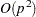bytes of working memory. Duringsuccessive iterations, uninterrupted by restarts or changes in the working set, the conjugate gradient algorithm computes a cycle ofconjugate search directions. In each iteration, a line search is performed along the search direction to find an approximate optimum of the objective function. The default line-search method uses quadratic interpolation and cubic extrapolation to obtain a step sizethat satisfies the Goldstein conditions. One of the Goldstein conditions can be violated if the feasible region defines an upper limit for the step size. Other line-search algorithms can be specified with the LIS= option.

The Nelder-Mead simplex method does not use any derivatives and does not assume that the objective function has continuous derivatives. The objective function itself needs to be continuous. This technique is quite expensive in the number of function calls, and it might be unable to generate precise results for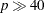.